# Sagemath¶

## Integration¶

Example 1: integration by parts

In :
show(integrate(2*pi*x*atan(x),x))


Example 2: trig sub

In :
show(integrate(x**2/sqrt(5 - 4*x**2),x))


Example 3: more integration by parts

In :
show(integrate(x**3*sin(x),x))


Example 4: trig sub with completing the square

In :
show(integrate(x*sqrt(x**2+2*x+4),x))


Example 5: integration of polynomials

In :
show(integrate(x * (x**2 + 5)**8, x))


Example 6: a trig integral

In :
show(integrate(sin(x)**5 * cos(x)**2,x))


Note: both ** and ^ can be used to indicate powers.

To see the help for a function, do:

In :
# ??integrate


## Polynomials and fractions¶

In :
A = expand((2*x+1)*(x-3)*(x**2+1))

In :
show(A)


This should give back the original product:

In :
factor(A)

Out:
(x^2 + 1)*(2*x + 1)*(x - 3)

Let's make a rational function:

In :
f(x)=(A/(x**2+4))


We can expand it into a sum:

In :
show(f.expand())


Or get the partial fraction decomposition:

In :
show(f.partial_fraction())


## Limits¶

Sage knows how to compute limits!

In :
limit(sin(x)/x, x= 0)

Out:
1
In :
limit((1-cos(x))/x**2, x=0)

Out:
1/2
In :
limit(x*log(x),x=0,dir='+')

Out:
0
In :
limit((1+x)**(1/x),x=0)

Out:
e
In :
limit(e^x/x,x=oo)

Out:
+Infinity

And occasionally it knows when a limit does not exist, too:

In :
limit(sin(1/x),x=0)

Out:
ind

This function becomes nasty close to $x=0$:

In :
plot(sin(1/x),x)

Out: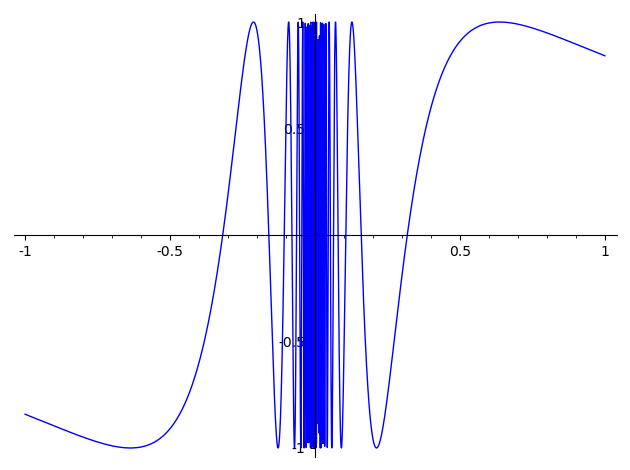## Orthogonal trajectories¶

Obtaining the equation for orthogonal trajectories for the given family of curves. First, we set up some variables and store the equation of our family into idvar

In :
k = var('k')
y = function('y')(x)
idvar = 4*x^2 + y^2 == k^2


The contents of idvar are exactly our equation. The == means we do not assign the right-hand side to the left-hand side, but rather compare them.

Let's differentiate both sides of this equality with respect to x:

In :
diff(idvar,x)

Out:
2*y(x)*diff(y(x), x) + 8*x == 0

The underscore _ stands for the previous output.

In :
solve(_,diff(y(x),x))

Out:
[diff(y(x), x) == -4*x/y(x)]

Let's extract the right-hand side in this list of one element. In Sage, as in Python, [a,b,c] denotes a list of the elements a, b, c.

In :
RHS = _.rhs()


Ok, now let's compute the negative inverse of the last output — that will give us the slope of orthogonal trajectories. Then, solve a differential equation with the o.t. slope in the right-hand side:

In :
desolve(diff(y,x) ==  -1/RHS, y, show_method=True)

Out:
[_C*x^(1/4), 'linear']

The following command will make several plots of the orthogonal trajectories for different value of the parameter _C. The plots are stored in the list ot_plots.

In :
ot_plots = [plot(_C*abs(x)^(1/4), color='magenta', xmin=-2, xmax=2, ymin=-2, ymax=2) for _C in [k*0.1 for k in [1..10]] ]


Make y a variable again for the plot.

In :
y = var('y')


Now let's make plots of the original curves. Instead of solving for y, we treat them as implicit plots.

In :
f(x,y,k)=4*x^2+y^2-k^2
orig_plots = [implicit_plot(4*x^2+y^2==k^2,(x,-2,2),(y,-2,2)) for k in srange(1,6,step=0.5)]


Now sum all the plots in the two lists we have built and show them:

In :
sum(orig_plots) + sum(ot_plots)

/usr/lib/python2.7/site-packages/matplotlib/contour.py:1230: UserWarning: No contour levels were found within the data range.
warnings.warn("No contour levels were found"

Out: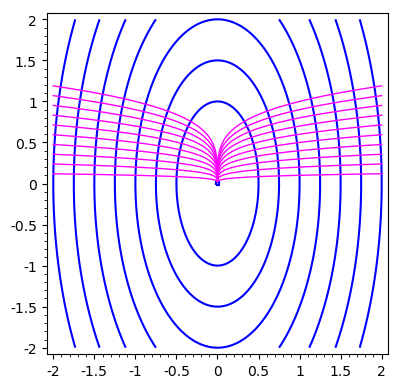The following is a pretty example in Maxima; Sage interface to Maxima seems buggy, as control seems to never go back to Sage?

In :
# maxima('plotdf(-2*x/y,[xfun,"sqrt(x);2*sqrt(x);sqrt(x);3*sqrt(x);-sqrt(x)"], [y,-10,10.1], [x,-10,10])$')  In any case, here is the same example using 'native' Sage: In : p1 = plot([sqrt(x),2*sqrt(x),sqrt(x),3*sqrt(x),-sqrt(x)], x, 0, 10) p2 = plot_vector_field((y,-2*x), (x,-10,10), (y,-10,10))  In : p1+p2  Out: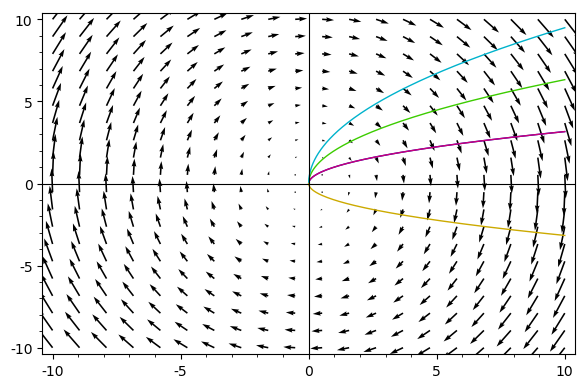## Plots¶ In : y = var('y')  In : implicit_plot(x^2 - y^2==0.1, (x,-2,2),(y,-2,2))  Out: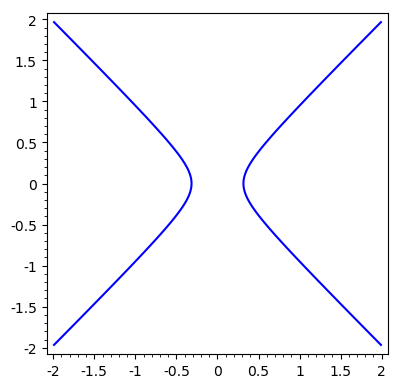In : polar_plot(sin(5*x)^2, (x, 0, 2*pi), color='blue')  Out: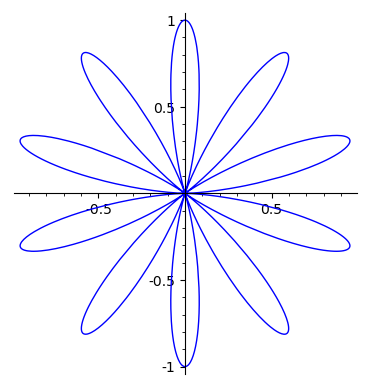## Differential equations¶ In : x = var('x') y = function('y')(x)  We will solve an example from the 2nd midterm here. In : desolve(diff(y,x)==2*x*sqrt(y), y, show_method=True)  Out: [sqrt(y(x)) == 1/2*x^2 + _C, 'separable'] Ok, so that gives us a generic solution of the equation; to incorporate the initial condition$ y(3) = 4 $, we use the ics keyword: In : deq = desolve(diff(y,x)==2*x*sqrt(y), y, ics=[3,4], show_method=True)  The answer becomes: In : deq  Out: [sqrt(y(x)) == 1/2*x^2 - 5/2, 'separable'] So we know the value of the constant as well. In order to obtain solution as an explicit function of$x$, we can solve the identity that is the first element of the list deq for y(x), as we did previously for the orthogonal trajectory. You can go ahead and try this: In : # solve(deq, y(x))  Sage will complain because it does not want to square both sides of this equation without knowing the sign of x^2 - 5. To fix this, let's make the following assumption: In : assume(x^2>5)  This now gives the answer we wanted: In : solve(deq, y(x))  Out: [y(x) == 1/4*x^4 - 5/2*x^2 + 25/4] Logistic model in Maxima (can freeze Sage indefinitely): In : # maxima('plotdf(0.08*P*(1-P/1e3), [t,P],[P,0,1400], [t,0,80], [xfun,"1000"])$')


Logistic with minimal population in Maxima (can freeze Sage indefinitely):

In :